QuestionWe need at least 10 more requests to produce the answer.

0 / 10 have requested this problem solution

The more requests, the faster the answer.

All students who have requested the answer will be notified once they are available.

#### Earn Coins

Coins can be redeemed for fabulous gifts.

Similar Homework Help Questions
• ### Estimate the crystal field stabilization energy for the octahedral ion hexacyanocobaltate(III), if the wavelength of maximum...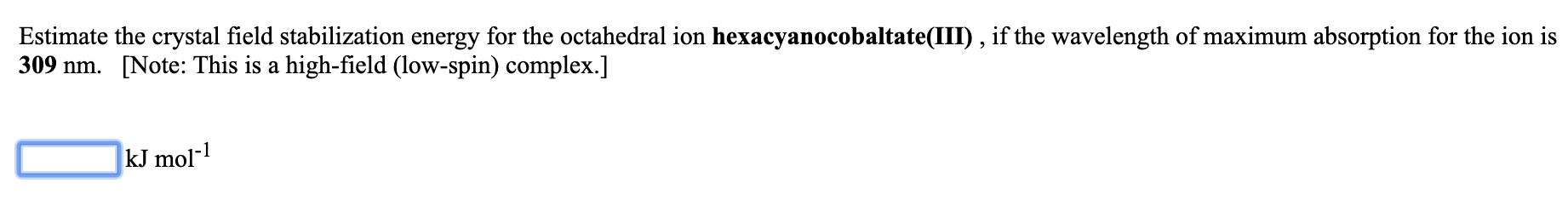Estimate the crystal field stabilization energy for the octahedral ion hexacyanocobaltate(III), if the wavelength of maximum absorption for the ion is 309 nm. [Note: This is a high-field (low-spin) complex.] kJ mol-1

• ### Estimate the crystal field stabilization energy for the octahedral ion hexafluorocobaltate(III), if the wavelength of maximum...

Estimate the crystal field stabilization energy for the octahedral ion hexafluorocobaltate(III), if the wavelength of maximum absorption for the ion is 710 nm.   [Note: This is a weak-field (high-spin) complex.] _____ kJ mol-1

• ### 13. An octahedral transition metal complex with a d' electron configuration absorbs light of wavelength equal to 650 nm. a. what is the magnitude of the octahedral crystal field splitting par...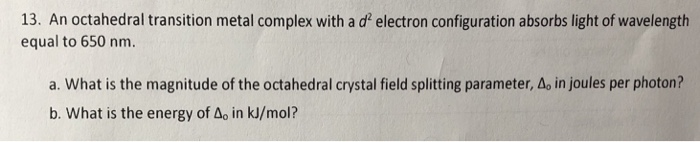13. An octahedral transition metal complex with a d' electron configuration absorbs light of wavelength equal to 650 nm. a. what is the magnitude of the octahedral crystal field splitting parameter, Δο in joules per photon? b. What is the energy of Ao in kJ/mol? 13. An octahedral transition metal complex with a d' electron configuration absorbs light of wavelength equal to 650 nm. a. what is the magnitude of the octahedral crystal field splitting parameter, Δο in joules per...

• ### 1) Estimate the crystal field stabilization energy for the octahedral ion hexaisothiocyanatotitanate(III), if the wavelength of...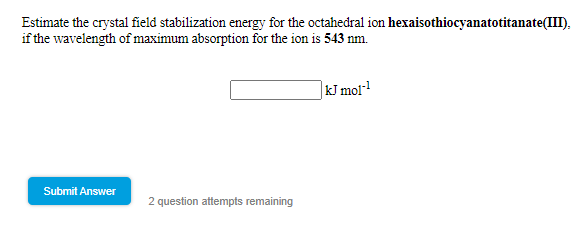1) Estimate the crystal field stabilization energy for the octahedral ion hexaisothiocyanatotitanate(III), if the wavelength of maximum absorption for the ion is 543 nm Estimate the crystal field stabilization energy for the octahedral ion hexaisothiocyanatotitanate(III). if the wavelength of maximum absorption for the ion is 543 nm. kJ moi? Submit Answer 2 question attempts remaining

• ### A ?1 octahedral complex is found to absorb visible light, with the absorption maximum occurring at 499 nm . Calculate the crystal-field splitting energy, Δ , in kJ/mol.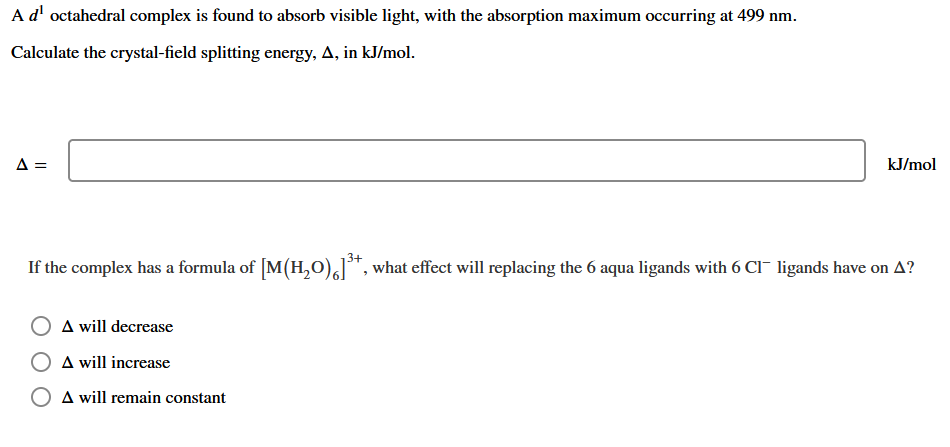A ?1 octahedral complex is found to absorb visible light, with the absorption maximum occurring at 499 nmCalculate the crystal-field splitting energy, Δin kJ/mol.

• ### Calculate the crystal field stabilization energy valiue for the complex Cr(NH)J*. The crystal field splitting energy...Calculate the crystal field stabilization energy valiue for the complex Cr(NH)J*. The crystal field splitting energy and pairing energy are found to be 150 kJ mol and 120 kJ mol respectively. 4.(c) 10 cc. Calculate the crystal field stabilization energy valiue for the complex Cr(NH)J*. The crystal field splitting energy and pairing energy are found to be 150 kJ mol and 120 kJ mol respectively. 4.(c) 10 cc.

• ### 620 nm Estimate the wavelength of maximum absorption for the octahedral ion trioxalatochromate(III) from the fact...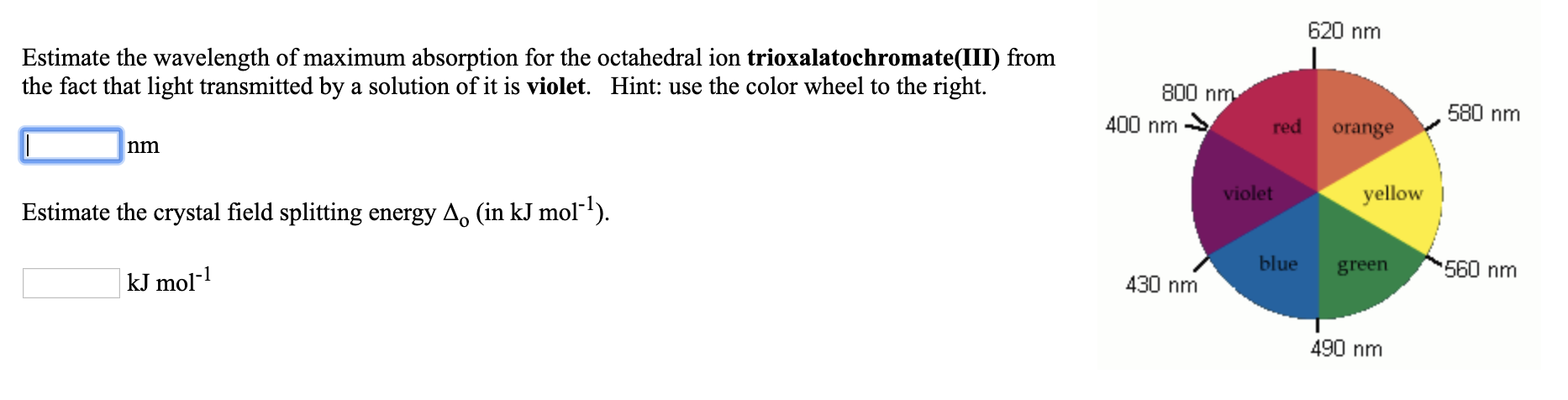620 nm Estimate the wavelength of maximum absorption for the octahedral ion trioxalatochromate(III) from the fact that light transmitted by a solution of it is violet. Hint: use the color wheel to the right. 800 nm 400 nm → red orange 580 nm violet yellow Estimate the crystal field splitting energy A. (in kJ mol-?). blue green kJ mol-1 560 nm 430 nm 490 nm

• ### Estimate the wavelength of maximum absorption for the octahedral ion hexaamminecobalt(lII) from right the fact that...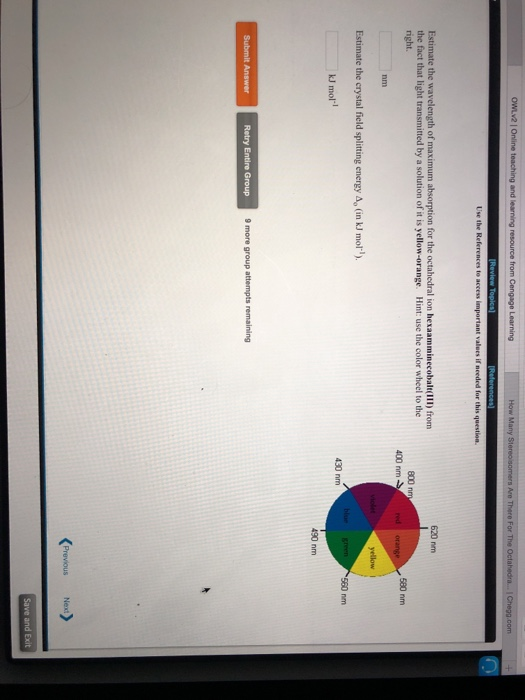Estimate the wavelength of maximum absorption for the octahedral ion hexaamminecobalt(lII) from right the fact that light transmitted by a solution of it is yellow-orange. Hint: use the color wheel to the 620 nm 800 nm 400 nm 580 nm nm Estimate the crystal field splitting energy Ap (in kJ mol) kJ mol-l 430 nm 490 nm Retry Entire Group 9 more group attempts remaining

• ### An octahedral metal complex absorbs light with wavelength 580 nm. a. What is the crystal field...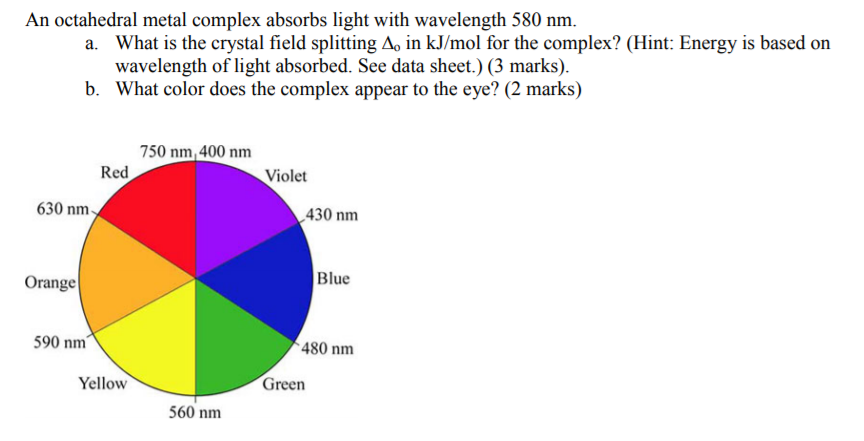An octahedral metal complex absorbs light with wavelength 580 nm. a. What is the crystal field splitting A, in kJ/mol for the complex? (Hint: Energy is based on wavelength of light absorbed. See data sheet.) (3 marks) b. What color does the complex appear to the eye? (2 marks) 750 nm, 400 nm Red Violet 630 nm 430 nm Blue Orange 590 nm 480 nm Yellow Green 560 nm

• ### The [CrCl_6]^3- ion has a maximum in its absorption spectrum at 735 nm. Calculate the crystal...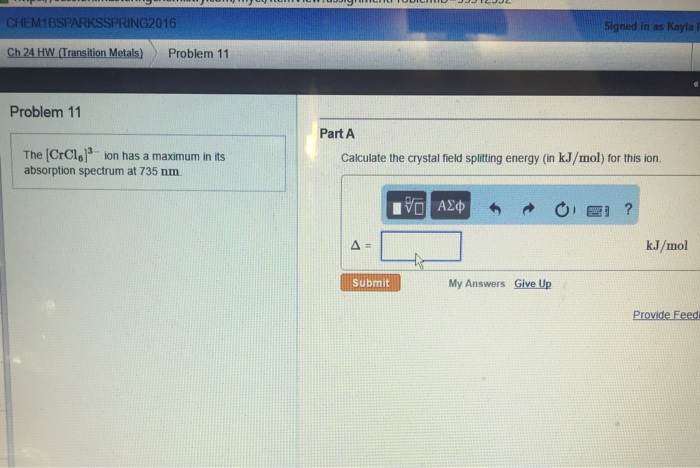The [CrCl_6]^3- ion has a maximum in its absorption spectrum at 735 nm. Calculate the crystal field splitting energy (in kJ/mol) for this ion.